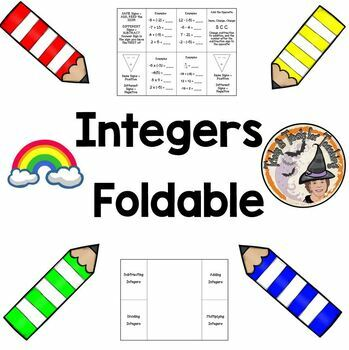Subject
Resource Type
File Type

Zip

(3 MB|3 pages)
Product Rating
Standards
• Product Description
• StandardsNEW

Teachers ~ Do you want an easy-to-use Foldable for your students to remember their Add Subtract Multiply and Divide Integers Rules? This is an INCREDIBLE product you'll LOVE using year after year! This is a ZIPFILE containing the Powerpoint version (fully editable) and the PDF version. You'll be able to edit the font, wording, examples, etc. to be tailor-made for your students! Plus you get the Answer KEY included. Enjoy!

Be sure to L@@K at my other, 675+ TERRIFIC teaching resources!

~ ~ THANK YOU KINDLY ~ ~

Apply properties of operations as strategies to multiply and divide rational numbers.
Understand that integers can be divided, provided that the divisor is not zero, and every quotient of integers (with non-zero divisor) is a rational number. If 𝘱 and 𝘲 are integers, then –(𝘱/𝘲) = (–𝘱)/𝘲 = 𝘱/(–𝘲). Interpret quotients of rational numbers by describing real-world contexts.
Understand that multiplication is extended from fractions to rational numbers by requiring that operations continue to satisfy the properties of operations, particularly the distributive property, leading to products such as (–1)(–1) = 1 and the rules for multiplying signed numbers. Interpret products of rational numbers by describing real-world contexts.
Apply and extend previous understandings of multiplication and division and of fractions to multiply and divide rational numbers.
Apply properties of operations as strategies to add and subtract rational numbers.
Total Pages
3 pages
Included
Teaching Duration
N/A
Report this Resource to TpT
Reported resources will be reviewed by our team. Report this resource to let us know if this resource violates TpT’s content guidelines.